2022 NL Rookie Odds
-110
0.91 to 1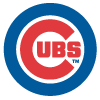Seiya Suzuki
34.6% implied probability

+400
4 to 1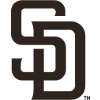MacKenzie Gore
13.2% implied probability

+800
8 to 1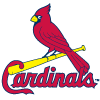Juan Yepez
7.3% implied probability

+1200
12 to 1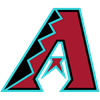Alek Thomas
5.1% implied probability

+2000
20 to 1Nolan Gorman
3.1% implied probability

+2000
20 to 1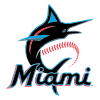Max Meyer
3.1% implied probability

+2500
25 to 1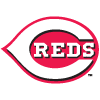Nick Lodolo
2.5% implied probability

+2500
25 to 1Oneil Cruz
2.5% implied probability

+3000
30 to 1Hunter Greene
2.1% implied probability

+3000
30 to 1CJ Abrams
2.1% implied probability

+4000
40 to 1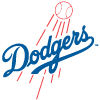Ryan Pepiot
1.6% implied probability

No changes have been recorded yet.
+4000
40 to 1Bryson Stott
1.6% implied probability

+4000
40 to 1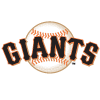Luis Gonzalez
1.6% implied probability

No changes have been recorded yet.
+4000
40 to 1Joey Bart
1.6% implied probability

+4000
40 to 1Seth Beer
1.6% implied probability

+4000
40 to 1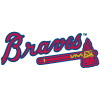Spencer Strider
1.6% implied probability

No changes have been recorded yet.
+6600
66 to 1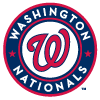Cade Cavalli
1.0% implied probability

+6600
66 to 1Brennen Davis
1.0% implied probability

+6600
66 to 1Geraldo Perdomo
1.0% implied probability

+8000
80 to 1Mickey Moniak
0.8% implied probability

+8000
80 to 1Roansy Contreras
0.8% implied probability

+8000
80 to 1Sixto Sanchez
0.8% implied probability

+8000
80 to 1Luis Campusano
0.8% implied probability

+8000
80 to 1Matt Vierling
0.8% implied probability

+10000
100 to 1Matthew Liberatore
0.7% implied probability

+10000
100 to 1Edward Cabrera
0.7% implied probability

+10000
100 to 1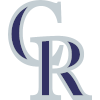Ryan Vilade
0.7% implied probability

+10000
100 to 1Heliot Ramos
0.7% implied probability

+12500
125 to 1Bobby Miller
0.5% implied probability

+15000
150 to 1Drew Waters
0.4% implied probability

+15000
150 to 1TJ Friedl
0.4% implied probability

+15000
150 to 1Ryan Rolison
0.4% implied probability

+15000
150 to 1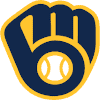Ethan Small
0.4% implied probability

+15000
150 to 1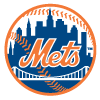Brett Baty
0.4% implied probability

+15000
150 to 1Mark Vientos
0.4% implied probability

+15000
150 to 1Ronny Mauricio
0.4% implied probability

+15000
150 to 1Brailyn Marquez
0.4% implied probability

+15000
150 to 1Nick Fortes
0.4% implied probability

+15000
150 to 1JJ Bleday
0.4% implied probability